# Bayesian time series intervention analysis¶

These are examples of using Bayesian statistics to infer the impact of an intervention whose effect follows one of several patterns mentioned in 9.2 Intervention Analysis:The calculations are done on PAWS, Wikimedia Cloud Services-hosted JupyterHub.

Update (29 Oct 2018): I've published the report/data analysis which motivated me to put together these notes.

## Autoregressive–moving-average model¶

For any ARMA model of observed series $y_t$ with change-due-to-intervention $z_t$ and error series $\epsilon_t$:

$$y_t = z_t + \frac{\Theta(B)}{\Phi(B)} \epsilon_t$$

where $\Theta(B)$ is the moving average (MA) polynomial and $\Phi(B)$ is the autoregressive (AR) polynomial.

In all of these, $z_t$ will be some function of an indicator variable $I_t$ and intervention effect $\delta_0$.

To keep these toy examples simple and easy to follow (at least with respect to simulation of data and performing the inference), we will use a stationary ARMA(1,1) model:

$$y_t = z_t + \phi_1 y_{t-1} + \theta_1 \epsilon_{t-1} + \epsilon_t$$

If you're seeing this time series stuff for the first time and are interested in learning more, I recommend the following resources:

## Inference¶

We are primarily interested in inference on the effect of the intervention $\delta_0$. We will use the the Stan probabilistic programming language to accomplish this, but others like JAGS, PyMC3, Edward2 / TensorFlow Probability, greta, Pyro, and Turing.jl can be used as well.

If you're seeing this Bayesian stuff for the first time and are interested in learning more, I recommend the following resources:

## Setup¶

We're using RStan to interface with Stan in R but all of this can also be done in Python with PyStan or in Julia with Stan.jl.

In :
library(magrittr)  # piping
library(zeallot)   # multi-assignment
library(tictoc)    # timing
library(latex2exp) # TeX to R expressions
suppressPackageStartupMessages(library(rstan))

In :
rstan_options(auto_write = TRUE)
options(
mc.cores = parallel::detectCores(),
repr.plot.width = 8, repr.plot.height = 4,
digits = 3
)


We will simulate $N = 200$ days of observations with intervention $T = 100$. The intervention effect is $\delta_0 = 10$ in all cases. In cases where there is a gradual increase/decrease, $\omega_1 = 0.9$.

The errors are i.i.d. from a Normal distribution ($\mu = 0, \sigma = 2$), and $\theta_1 = 0.6$ and $\phi_1 = -0.7$ as the MA(1) and AR(1) coefficients, respectively:

In :
c(N, T, theta1, phi1, delta0, sigma, omega1) %<-% c(200, 100, 0.6, -0.7, 10, 2, 0.9)

# Helper function to simulate an ARMA(1,1) series which includes changes from intervention:
arma11_sim <- function(z) {
# Setup:
y <- numeric(0)
set.seed(0)
y <- rnorm(1, 0, 5)

# Stochastic component:
errors <- c(0, rnorm(N - 1, 0, sd = sigma))

# Deterministic component:
for (t in 2:N) y[t] <- z[t] + phi1 * y[t - 1] + theta1 * errors[t - 1] + errors[t]

# Output:
return(y)
}

# Helper functions to plot time series and visualize the change from intervention:
plot_change <- function(z, highlight_idx) {
plot(z, type = "l", ylab = expression(z[t]), xlab = expression(t), main = "Change due to intervention")
highlight_idx <- c(highlight_idx - 1, highlight_idx, highlight_idx[length(highlight_idx)] + 1)
lines(highlight_idx, z[highlight_idx], col = "red")
}
plot_ts <- function(y, z, highlight_idx) {
par(mfrow = c(1, 2))
plot(y, type = "l", ylab = expression(y[t]), xlab = expression(t), main = "Observed (simulated) series")
plot_change(z, highlight_idx)
}

# Helper function to compare point estimates & credible intervals from posterior to the truth:
compare <- function(stan_fit, actual, ...) {
fit_summary <- stan_fit %>%
broom::tidyMCMC(estimate.method = "median", conf.int = TRUE, conf.method = "HPDinterval", ...) %>%
dplyr::mutate(conf.95 = sprintf("(%0.2f, %0.2f)", conf.low, conf.high)) %>%
dplyr::select(-c(conf.low, conf.high))
return(cbind(fit_summary, actual = actual))
}


## Patterns¶

### Pattern 1: constant permanent change¶

If $T$ is the time of intervention, then $I_t = 0$ when $t < T$ and $I_t = 1$ when $t \geq T$.

$$z_t = \delta_0 I_t$$
In :
I_1 <- (1:N) >= T
z_1 <- delta0 * I_1

y_1 <- arma11_sim(z_1)
plot_ts(y_1, z_1, which(I_1))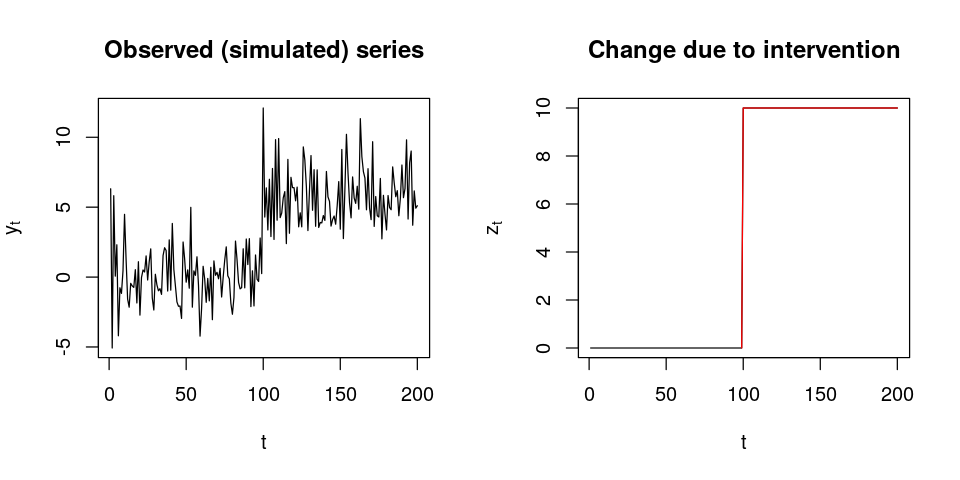The Stan manual authors recommend constraining the coefficients to enforce the estimation of a stationary ARMA process so that's what we're going to do here. Although they don't recommended that in practice in case the data is not stationary, in which case they suggest encouraging stationary parameter estimates with a prior favoring coefficient values near zero.

In :
model_1 <- stan_model(model_name = "pattern_1", model_code = "
data {
int<lower = 1> T; // known time of intervention
int<lower = 1> N; // number observations in series
real y[N];        // observations
}
parameters {
real<lower = -1, upper = 1> phi1;
real<lower = -1, upper = 1> theta1;
real delta0;
real<lower = 0> sigma;
}
model {
vector[N] nu;      // prediction at time t
vector[N] z;       // effect of intervention at t
vector[N] epsilon; // diff between pred & obs at t

// priors:
phi1 ~ normal(0, 1);
theta1 ~ normal(0, 1);
delta0 ~ normal(0, 5);
sigma ~ cauchy(0, 5);

// likelihood:
z = 0;
epsilon = 0;
for (t in 2:N) {
z[t] = delta0 * (t >= T ? 1 : 0);
nu[t] = (phi1 * y[t - 1]) + (theta1 * epsilon[t - 1]) + z[t];
epsilon[t] = y[t] - nu[t];
}
epsilon ~ normal(0, sigma);
}")


Once we have a model we can do Bayesian inference in one of two ways:

Let's see how the results differ and how long they take to get:

In :
tic()
fit_1a <- vb(model_1, data = list(T = T, N = N, y = y_1))
toc()
compare(fit_1a, c(phi1, theta1, delta0, sigma), pars = c("phi1", "theta1", "delta0", "sigma"))

Chain 1: ------------------------------------------------------------
Chain 1: EXPERIMENTAL ALGORITHM:
Chain 1:   This procedure has not been thoroughly tested and may be unstable
Chain 1:   or buggy. The interface is subject to change.
Chain 1: ------------------------------------------------------------
Chain 1:
Chain 1:
Chain 1:
Chain 1: Gradient evaluation took 7.4e-05 seconds
Chain 1: 1000 transitions using 10 leapfrog steps per transition would take 0.74 seconds.
Chain 1:
Chain 1:
Chain 1: Iteration:   1 / 250 [  0%]  (Adaptation)
Chain 1: Iteration:  50 / 250 [ 20%]  (Adaptation)
Chain 1: Iteration: 100 / 250 [ 40%]  (Adaptation)
Chain 1: Iteration: 150 / 250 [ 60%]  (Adaptation)
Chain 1: Iteration: 200 / 250 [ 80%]  (Adaptation)
Chain 1: Success! Found best value [eta = 1] earlier than expected.
Chain 1:
Chain 1: Begin stochastic gradient ascent.
Chain 1:   iter             ELBO   delta_ELBO_mean   delta_ELBO_med   notes
Chain 1:    100         -461.856             1.000            1.000
Chain 1:    200         -432.881             0.533            1.000
Chain 1:    300         -427.209             0.360            0.067
Chain 1:    400         -424.674             0.272            0.067
Chain 1:    500         -421.651             0.219            0.013
Chain 1:    600         -420.765             0.183            0.013
Chain 1:    700         -421.169             0.157            0.007   MEDIAN ELBO CONVERGED
Chain 1:
Chain 1: Drawing a sample of size 1000 from the approximate posterior...
Chain 1: COMPLETED.
0.271 sec elapsed

termestimatestd.errorconf.95actual
phi1 -0.749 0.0361 (-0.81, -0.67)-0.7
theta1 0.670 0.0681 (0.53, 0.79) 0.6
delta0 10.246 0.2821 (9.71, 10.80) 10.0
sigma 1.830 0.0942 (1.65, 2.03) 2.0
In :
tic()
fit_1b <- sampling(model_1, data = list(T = T, N = N, y = y_1))
toc()
compare(fit_1b, c(phi1, theta1, delta0, sigma), pars = c("phi1", "theta1", "delta0", "sigma"))

0.991 sec elapsed

termestimatestd.errorconf.95actual
phi1 -0.742 0.0771 (-0.87, -0.58)-0.7
theta1 0.651 0.1102 (0.43, 0.84) 0.6
delta0 10.095 0.5258 (9.00, 11.03) 10.0
sigma 1.849 0.0935 (1.67, 2.04) 2.0

Pretty good results in way less time!

The original draft of this notebook just had MCMC and it wasn't until right before I was ready to post it that I was like "let's just try VI and see what happens". Since it's not expensive to do it in addition to the full inference, I've included in the other patterns too to show its similarities, differences, advantages, and shortcomings. I feel like approximate inference with (AD)VI isn't as well-known among Bayesian statisticians and data scientists as MCMC-powered full inference is, so hopefully this could be a cool introduction to that.

### Pattern 2: temporary, constant change¶

When the change from the intervention lasts $d$ days, $I_t = 1$ for $T \leq t \leq T + d$ and $I_t = 0$ for all other $t$.

$$z_t = \delta_0 I_t$$
In :
d = 18
I_2 <- ((1:N) >= T) & ((1:N) <= (T + d))
z_2 <- delta0 * I_2

y_2 <- arma11_sim(z_2)
plot_ts(y_2, z_2, which(I_2))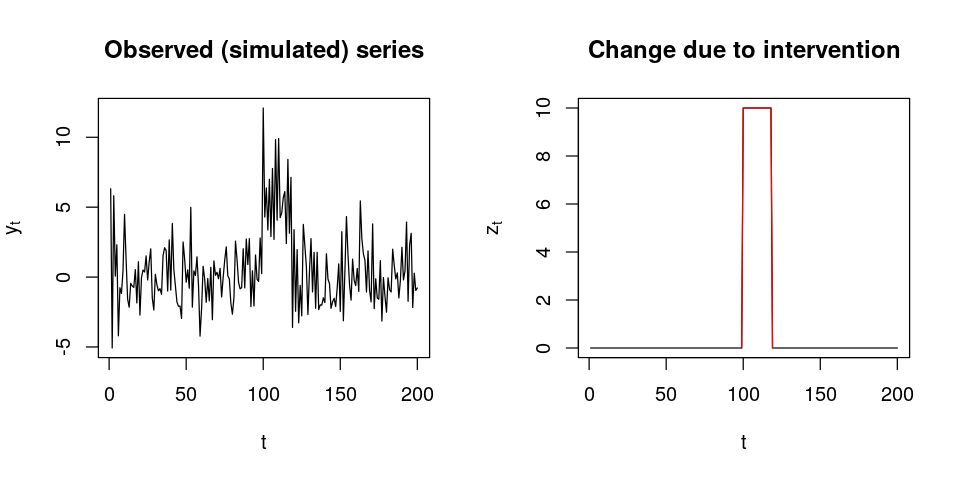In :
model_2 <- stan_model(model_name = "pattern_2", model_code = "
data {
int<lower = 1> T; // known time of intervention
int<lower = 1> d; // days the change from intervention lasts
int<lower = 1> N; // number observations in series
real y[N];        // observations
}
parameters {
real<lower = -1, upper = 1> phi1;
real<lower = -1, upper = 1> theta1;
real delta0;
real<lower = 0> sigma;
}
model {
vector[N] nu;      // prediction at time t
vector[N] z;       // effect of intervention at t
vector[N] epsilon; // diff between pred & obs at t

// priors:
phi1 ~ normal(0, 1);
theta1 ~ normal(0, 1);
delta0 ~ normal(0, 5);
sigma ~ cauchy(0, 5);

// likelihood:
z = 0;
epsilon = 0;
for (t in 2:N) {
z[t] = delta0 * (((t >= T) && (t <= (T + d))) ? 1 : 0);
nu[t] = (phi1 * y[t - 1]) + (theta1 * epsilon[t - 1]) + z[t];
epsilon[t] = y[t] - nu[t];
}
epsilon ~ normal(0, sigma);
}")

In :
tic()
fit_2a <- vb(model_2, data = list(T = T, N = N, y = y_2, d = d))
toc()
compare(fit_2a, c(phi1, theta1, delta0, sigma), pars = c("phi1", "theta1", "delta0", "sigma"))

Chain 1: ------------------------------------------------------------
Chain 1: EXPERIMENTAL ALGORITHM:
Chain 1:   This procedure has not been thoroughly tested and may be unstable
Chain 1:   or buggy. The interface is subject to change.
Chain 1: ------------------------------------------------------------
Chain 1:
Chain 1:
Chain 1:
Chain 1: Gradient evaluation took 6.6e-05 seconds
Chain 1: 1000 transitions using 10 leapfrog steps per transition would take 0.66 seconds.
Chain 1:
Chain 1:
Chain 1: Iteration:   1 / 250 [  0%]  (Adaptation)
Chain 1: Iteration:  50 / 250 [ 20%]  (Adaptation)
Chain 1: Iteration: 100 / 250 [ 40%]  (Adaptation)
Chain 1: Iteration: 150 / 250 [ 60%]  (Adaptation)
Chain 1: Iteration: 200 / 250 [ 80%]  (Adaptation)
Chain 1: Success! Found best value [eta = 1] earlier than expected.
Chain 1:
Chain 1: Begin stochastic gradient ascent.
Chain 1:   iter             ELBO   delta_ELBO_mean   delta_ELBO_med   notes
Chain 1:    100         -439.923             1.000            1.000
Chain 1:    200         -422.301             0.521            1.000
Chain 1:    300         -419.749             0.349            0.042
Chain 1:    400         -419.996             0.262            0.042
Chain 1:    500         -420.866             0.210            0.006   MEDIAN ELBO CONVERGED
Chain 1:
Chain 1: Drawing a sample of size 1000 from the approximate posterior...
Chain 1: COMPLETED.
0.299 sec elapsed

termestimatestd.errorconf.95actual
phi1 -0.760 0.0393 (-0.83, -0.68)-0.7
theta1 0.661 0.0594 (0.54, 0.77) 0.6
delta0 10.138 0.6351 (8.90, 11.37) 10.0
sigma 1.725 0.0904 (1.57, 1.92) 2.0
In :
tic()
fit_2b <- sampling(model_2, data = list(T = T, N = N, y = y_2, d = d), control = list(adapt_delta = 0.9))
toc()
compare(fit_2b, c(phi1, theta1, delta0, sigma), pars = c("phi1", "theta1", "delta0", "sigma"))

1.351 sec elapsed

termestimatestd.errorconf.95actual
phi1 -0.749 0.0620 (-0.85, -0.61)-0.7
theta1 0.659 0.0976 (0.46, 0.83) 0.6
delta0 9.829 0.7026 (8.40, 11.17) 10.0
sigma 1.845 0.0947 (1.67, 2.05) 2.0

### Pattern 3: gradually leveling-off change¶

If $T$ is the time of intervention, then $I_t = 0$ when $t < T$ and $I_t = 1$ when $t \geq T$.

$$z_t = \frac{\delta_0}{1 - \omega_1 B} I_t$$

assuming $\omega_1 < 1$. The above is equivalent to $z_t = \omega_1 z_{t-1} + \delta_0 I_t$. Furthermore, since $|\omega_1| < 1$, $z_t$ can be expressed just in terms of $\omega_1$ and $\delta_0$:

$$z_t = \frac{\delta_0(1 - \omega_1^{t - T + 1})}{1 - \omega_1}$$
In :
z_3 <- numeric(N)
for (t in 2:N) z_3[t] <- omega1 * z_3[t - 1] + delta0 * (t >= T)

y_3 <- arma11_sim(z_3)
plot_ts(y_3, z_3, which((1:N) >= T))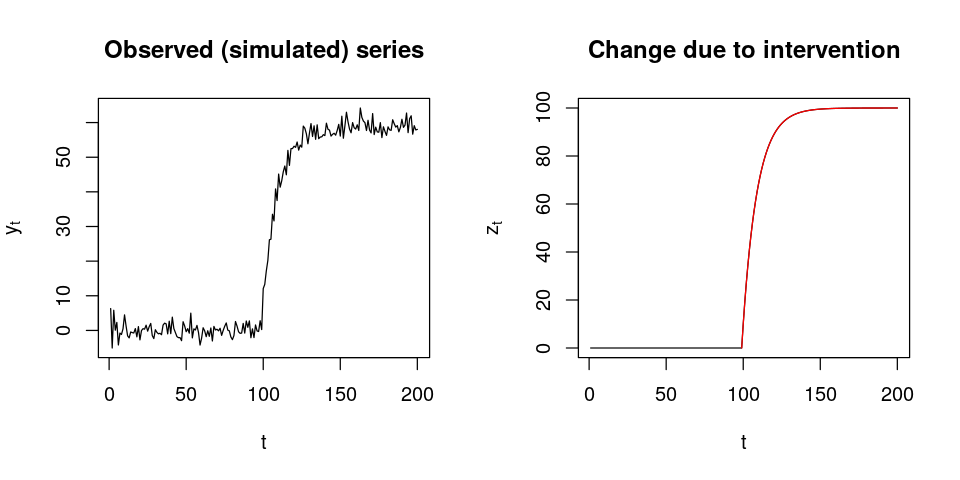Knowing how the value of $\omega_1$ affects the effect can help us come up with an informative prior for it:

In :
zs <- numeric(N)
omegas <- c(0.1, 0.5, 0.7, 0.8, 0.9)
plot(1, type = "n", xlim = c(95, 160), ylim = c(0, 10), yaxt = "n",
xlab = "t", ylab = expression(z[t]),
main = expression(paste("How ", omega, " influences effect")))
for (o in seq_along(omegas)) {
for (t in 2:N) zs[t] <- omegas[o] * zs[t - 1] + 1 * (t >= T)
lines(95:155, zs[95:155], type = "l")
text(155, zs, omegas[o], pos = 4)
}; rm(zs, omegas, o, t)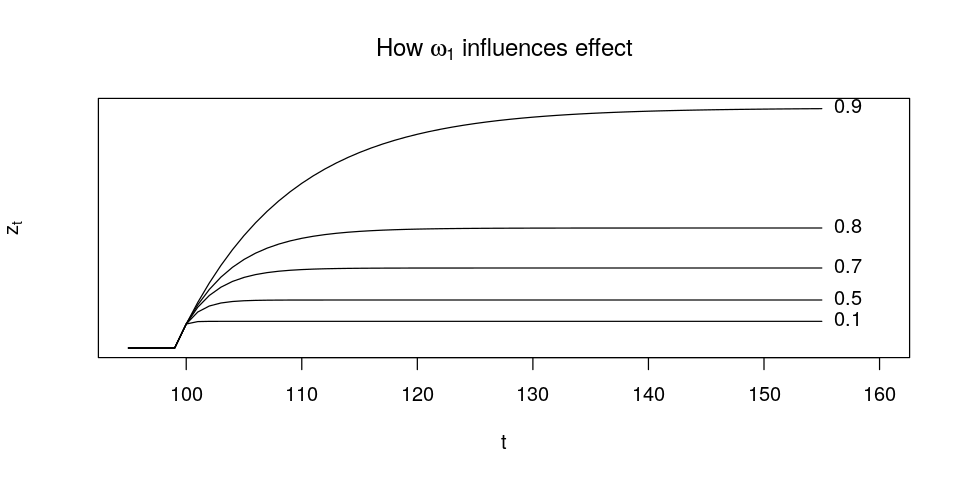Looking at the observed $y_t$, it appears that it takes about 50 days before the effect stops increasing. We don't need to know $\delta_0$ to see how $z_t$ behaves under different values of $\omega_1$. Looking at the figure above, we could be confident in saying that $\omega_1$ is definitely bigger than 0.1 and probably bigger than 0.5. Instead of a Uniform(0, 1) prior, it looks like we would be wise to use a Beta(8, 2) prior instead, which gives values 0.7-0.9 much higher probability:In :
model_3 <- stan_model(model_name = "pattern_3", model_code = "
data {
int<lower = 1> T; // known time of intervention
int<lower = 1> N; // number observations in series
real y[N];        // observations
}
parameters {
real<lower = -1, upper = 1> phi1;
real<lower = -1, upper = 1> theta1;
real<lower = 0> delta0;
real<lower = 0, upper = 1> omega1;
real<lower = 0> sigma;
}
model {
vector[N] nu;      // prediction at time t
vector[N] z;       // effect of intervention at t
vector[N] epsilon; // diff between pred & obs at t

// priors:
phi1 ~ normal(0, 1);
theta1 ~ normal(0, 1);
delta0 ~ normal(5, 5);
sigma ~ cauchy(0, 5);
omega1 ~ beta(8, 2);

// likelihood:
z = 0;
epsilon = 0;
for (t in 2:N) {
if (t < T) {
z[t] = 0;
} else {
z[t] = delta0 * (1 - (omega1 ^ (t - T + 1))) / (1 - omega1);
}
nu[t] = (phi1 * y[t - 1]) + (theta1 * epsilon[t - 1]) + z[t];
epsilon[t] = y[t] - nu[t];
}
epsilon ~ normal(0, sigma);
}")

In :
tic()
fit_3a <- vb(model_3, data = list(T = T, N = N, y = y_3))
toc()
compare(fit_3a, c(phi1, theta1, delta0, sigma, omega1), pars = c("phi1", "theta1", "delta0", "sigma", "omega1"))

Chain 1: ------------------------------------------------------------
Chain 1: EXPERIMENTAL ALGORITHM:
Chain 1:   This procedure has not been thoroughly tested and may be unstable
Chain 1:   or buggy. The interface is subject to change.
Chain 1: ------------------------------------------------------------
Chain 1:
Chain 1:
Chain 1:
Chain 1: Gradient evaluation took 0.0001 seconds
Chain 1: 1000 transitions using 10 leapfrog steps per transition would take 1 seconds.
Chain 1:
Chain 1:
Chain 1: Iteration:   1 / 250 [  0%]  (Adaptation)
Chain 1: Iteration:  50 / 250 [ 20%]  (Adaptation)
Chain 1: Iteration: 100 / 250 [ 40%]  (Adaptation)
Chain 1: Iteration: 150 / 250 [ 60%]  (Adaptation)
Chain 1: Iteration: 200 / 250 [ 80%]  (Adaptation)
Chain 1: Success! Found best value [eta = 1] earlier than expected.
Chain 1:
Chain 1: Begin stochastic gradient ascent.
Chain 1:   iter             ELBO   delta_ELBO_mean   delta_ELBO_med   notes
Chain 1:    100         -743.089             1.000            1.000
Chain 1:    200         -515.682             0.720            1.000
Chain 1:    300         -489.919             0.498            0.441
Chain 1:    400         -480.154             0.378            0.441
Chain 1:    500         -488.239             0.306            0.053
Chain 1:    600         -473.757             0.260            0.053
Chain 1:    700         -481.765             0.225            0.031
Chain 1:    800         -471.077             0.200            0.031
Chain 1:    900         -473.603             0.178            0.023
Chain 1:   1000         -471.869             0.161            0.023
Chain 1:   1100         -470.562             0.061            0.020
Chain 1:   1200         -473.105             0.018            0.017
Chain 1:   1300         -471.327             0.013            0.017
Chain 1:   1400         -496.985             0.016            0.017
Chain 1:   1500         -475.814             0.019            0.017
Chain 1:   1600         -469.199             0.017            0.014
Chain 1:   1700         -477.333             0.017            0.014
Chain 1:   1800         -468.517             0.017            0.014
Chain 1:   1900         -476.607             0.018            0.017
Chain 1:   2000         -491.647             0.021            0.017
Chain 1:   2100         -467.207             0.026            0.019
Chain 1:   2200         -469.900             0.026            0.019
Chain 1:   2300         -495.053             0.030            0.031
Chain 1:   2400         -466.618             0.031            0.031
Chain 1:   2500         -475.035             0.029            0.019
Chain 1:   2600         -469.141             0.028            0.019
Chain 1:   2700         -467.407             0.027            0.019
Chain 1:   2800         -471.520             0.026            0.018
Chain 1:   2900         -467.019             0.025            0.018
Chain 1:   3000         -468.643             0.023            0.013
Chain 1:   3100         -467.173             0.018            0.010   MEDIAN ELBO CONVERGED
Chain 1:
Chain 1: Drawing a sample of size 1000 from the approximate posterior...
Chain 1: COMPLETED.
0.36 sec elapsed

termestimatestd.errorconf.95actual
phi1 0.888 0.00132 (0.88, 0.89) -0.7
theta1 -0.681 0.05430 (-0.78, -0.57) 0.6
delta0 3.991 0.04587 (3.91, 4.08) 10.0
sigma 2.331 0.11997 (2.11, 2.56) 2.0
omega1 0.397 0.00767 (0.38, 0.41) 0.9
In :
tic()
fit_3b <- sampling(model_3, data = list(T = T, N = N, y = y_3),
iter = 40000, control = list(max_treedepth = 15, adapt_delta = 0.999))
toc()
compare(fit_3b, c(phi1, theta1, delta0, sigma, omega1), pars = c("phi1", "theta1", "delta0", "sigma", "omega1"))

Warning message:
“There were 1 chains where the estimated Bayesian Fraction of Missing Information was low. See
http://mc-stan.org/misc/warnings.html#bfmi-low”Warning message:
“Examine the pairs() plot to diagnose sampling problems
”
784.288 sec elapsed

termestimatestd.errorconf.95actual
phi1 -0.737 0.2056 (-0.88, -0.54)-0.7
theta1 0.640 0.1935 (0.39, 0.83) 0.6
delta0 10.094 0.8574 (8.96, 11.03) 10.0
sigma 1.856 0.1033 (1.67, 2.06) 2.0
omega1 0.901 0.0609 (0.90, 0.91) 0.9

### Pattern 4: immediate, gradually decreasing change¶

The formula and model specification are the same as the pattern above except $I_t = 1$ when $t = T$ and $I_t = 0$ otherwise.

In :
z_4 <- numeric(N)
for (t in 2:N) z_4[t] <- omega1 * z_4[t - 1] + delta0 * (t == T)

y_4 <- arma11_sim(z_4)
plot_ts(y_4, z_4, which((1:N) >= T))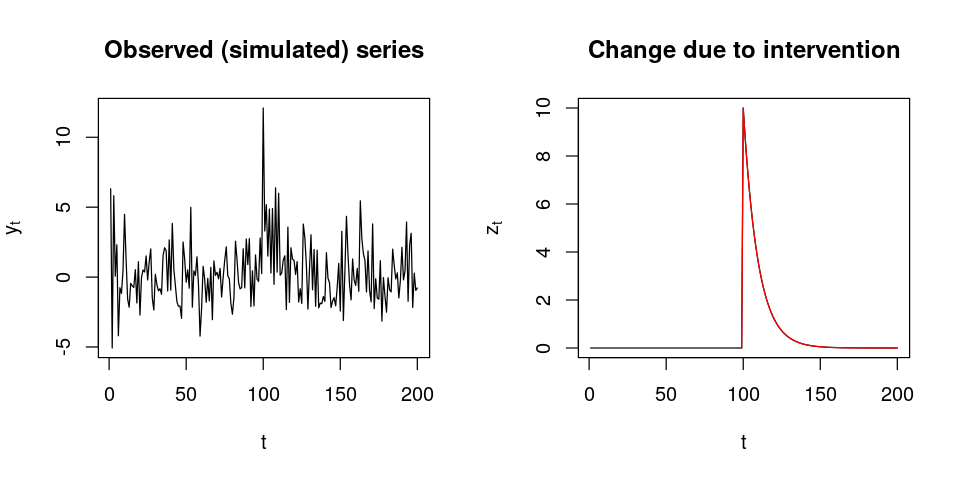In :
model_4 <- stan_model(model_name = "pattern_4", model_code = "
data {
int<lower = 1> T; // known time of intervention
int<lower = 1> N; // number observations in series
real y[N];        // observations
}
parameters {
real<lower = -1, upper = 1> phi1;
real<lower = -1, upper = 1> theta1;
real<lower = 0> delta0;
real<lower = 0, upper = 1> omega1;
real<lower = 0> sigma;
}
model {
vector[N] nu;      // prediction at time t
vector[N] z;       // effect of intervention at t
vector[N] epsilon; // diff between pred & obs at t

// priors:
phi1 ~ normal(0, 1);
theta1 ~ normal(0, 1);
delta0 ~ normal(0, 5);
sigma ~ cauchy(0, 5);
omega1 ~ beta(6, 2);

// likelihood:
z = 0;
epsilon = 0;
for (t in 2:N) {
z[t] = omega1 * z[t - 1] + delta0 * (t == T ? 1 : 0);
nu[t] = (phi1 * y[t - 1]) + (theta1 * epsilon[t - 1]) + z[t];
epsilon[t] = y[t] - nu[t];
}
epsilon ~ normal(0, sigma);
}")

In :
tic()
fit_4a <- vb(model_4, data = list(T = T, N = N, y = y_4))
toc()
compare(fit_4a, c(phi1, theta1, delta0, sigma, omega1), pars = c("phi1", "theta1", "delta0", "sigma", "omega1"))

Chain 1: ------------------------------------------------------------
Chain 1: EXPERIMENTAL ALGORITHM:
Chain 1:   This procedure has not been thoroughly tested and may be unstable
Chain 1:   or buggy. The interface is subject to change.
Chain 1: ------------------------------------------------------------
Chain 1:
Chain 1:
Chain 1:
Chain 1: Gradient evaluation took 8.8e-05 seconds
Chain 1: 1000 transitions using 10 leapfrog steps per transition would take 0.88 seconds.
Chain 1:
Chain 1:
Chain 1: Iteration:   1 / 250 [  0%]  (Adaptation)
Chain 1: Iteration:  50 / 250 [ 20%]  (Adaptation)
Chain 1: Iteration: 100 / 250 [ 40%]  (Adaptation)
Chain 1: Iteration: 150 / 250 [ 60%]  (Adaptation)
Chain 1: Iteration: 200 / 250 [ 80%]  (Adaptation)
Chain 1: Iteration: 250 / 250 [100%]  (Adaptation)
Chain 1: Success! Found best value [eta = 0.1].
Chain 1:
Chain 1: Begin stochastic gradient ascent.
Chain 1:   iter             ELBO   delta_ELBO_mean   delta_ELBO_med   notes
Chain 1:    100         -482.381             1.000            1.000
Chain 1:    200         -467.389             0.516            1.000
Chain 1:    300         -461.836             0.348            0.032
Chain 1:    400         -459.217             0.262            0.032
Chain 1:    500         -465.523             0.213            0.014
Chain 1:    600         -477.843             0.182            0.026
Chain 1:    700         -457.058             0.162            0.026
Chain 1:    800         -452.213             0.143            0.026
Chain 1:    900         -463.127             0.130            0.024
Chain 1:   1000         -452.065             0.119            0.024
Chain 1:   1100         -451.591             0.019            0.024
Chain 1:   1200         -453.756             0.017            0.014
Chain 1:   1300         -451.621             0.016            0.014
Chain 1:   1400         -450.407             0.016            0.014
Chain 1:   1500         -536.885             0.030            0.024
Chain 1:   1600         -451.569             0.047            0.024
Chain 1:   1700         -454.922             0.043            0.011
Chain 1:   1800         -453.118             0.042            0.007   MEDIAN ELBO CONVERGED
Chain 1:
Chain 1: Drawing a sample of size 1000 from the approximate posterior...
Chain 1: COMPLETED.
0.238 sec elapsed

termestimatestd.errorconf.95actual
phi1 0.0998 0.0771 (-0.06, 0.24)-0.7
theta1 -0.1270 0.0652 (-0.25, 0.01) 0.6
delta0 0.9813 4.8850 (0.01, 9.88) 10.0
sigma 2.1833 0.1239 (1.94, 2.43) 2.0
omega1 0.7861 0.1314 (0.51, 0.96) 0.9
In :
tic()
fit_4b <- sampling(model_4, data = list(T = T, N = N, y = y_4),
iter = 10000, control = list(max_treedepth = 20, adapt_delta = 0.99))
toc()
compare(fit_4b, c(phi1, theta1, delta0, sigma, omega1), pars = c("phi1", "theta1", "delta0", "sigma", "omega1"))

9.477 sec elapsed

termestimatestd.errorconf.95actual
phi1 -0.733 0.0840 (-0.86, -0.55)-0.7
theta1 0.637 0.1172 (0.40, 0.84) 0.6
delta0 10.323 1.4398 (7.60, 13.18) 10.0
sigma 1.851 0.0947 (1.68, 2.04) 2.0
omega1 0.877 0.0311 (0.81, 0.93) 0.9

### Pattern 5: Gompertz (sigmoidal)¶

If there's a slow ramp-up and then the change slowly levels-off in an "S" shape, we can represent it with the Gompertz function:

$$f(t) = a\mathrm{e}^{-b\mathrm{e}^{-ct}}$$

where

• $a$ is an asymptote (e.g. $\delta_0$), since $\lim_{t \to \infty} a\mathrm{e}^{-b\mathrm{e}^{-ct }}=a\mathrm{e}^0=a$
• $b > 0$ sets the displacement along the x-axis (translates the graph to the left or right)
• $c > 0$ sets the growth rate (we'll call it $\lambda$)
• e is Euler's Number)
In :
gompertz <- function(t, a, b, c) {
return(a * exp(-b * exp(-c * t)))
}

In :
zs <- numeric(N)
lambdas <- c(0.25, 0.5, 1, 1.5)
plot(1, type = "n", xlim = c(95, 130), ylim = c(0, delta0),# yaxt = "n",
xlab = "t", ylab = expression(z[t]),
main = TeX("How $\\lambda$ influences effect"))
for (l in seq_along(lambdas)) {
for (t in 2:N) {
if (t >= T) {
zs[t] <- gompertz(t - T, delta0, d, lambdas[l])
} else {
zs[t] <- 0
}
}
lines(95:130, zs[95:130], type = "l", col = length(lambdas) + 1 - l, lty = l, lwd = 1.5)
}; rm(zs, l, t)
legend("bottomright", legend = lambdas,
lwd = 1.5, lty = 1:length(lambdas),
col = length(lambdas) + 1 - (1:length(lambdas)),
y.intersp = 3, bty = "n")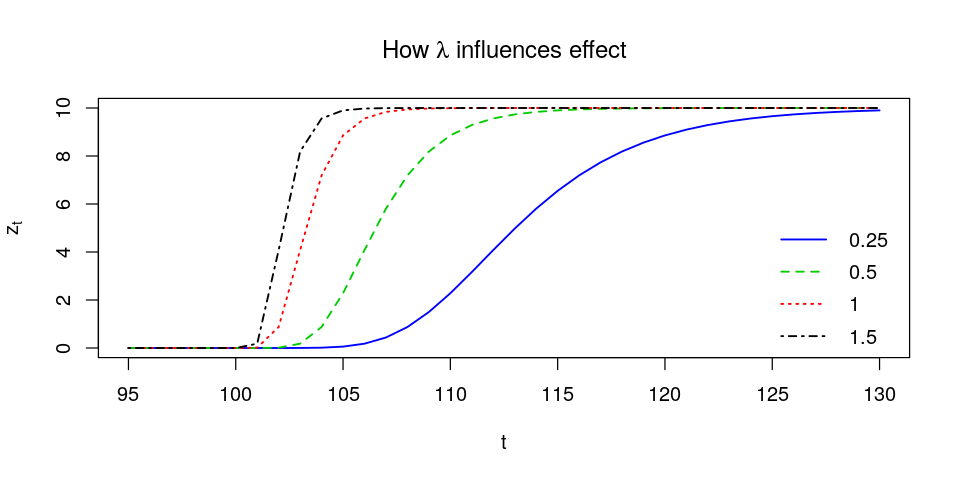In :
I_5 <- (1:N) >= T
lambda <- 0.1
z_5 <- gompertz((1:N) - T, delta0, d, lambda) * I_5

y_5 <- arma11_sim(z_5)
plot_ts(y_5, z_5, which(I_5))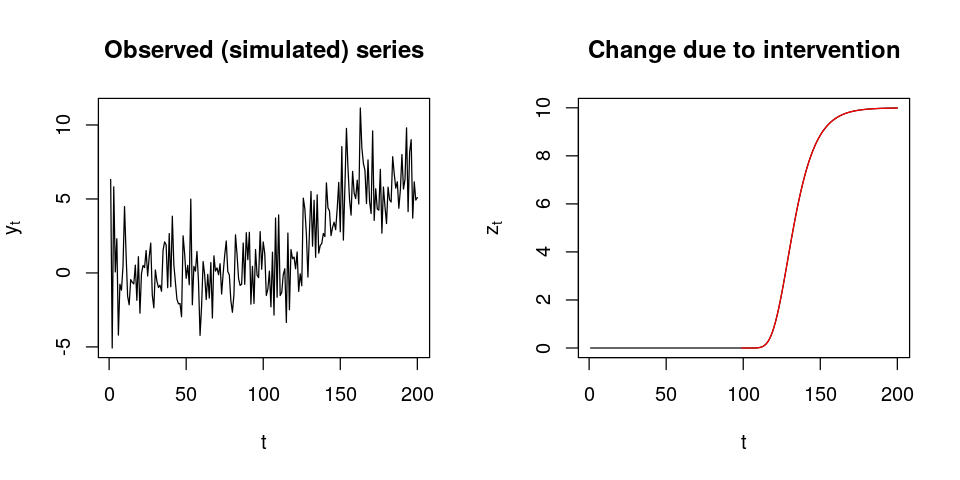In :
model_5 <- stan_model(model_name = "pattern_5", model_code = "
functions {
real gompertz(real t, real a, real b, real c) {
return (a * exp(-b * exp(-c * t)));
}
}
data {
int<lower = 1> T; // known time of intervention
int<lower = 1> N; // number observations in series
real y[N];        // observations
int<lower = 1> d; // midpoint/displacement along t
}
parameters {
real<lower = -1, upper = 1> phi1;
real<lower = -1, upper = 1> theta1;
real<lower = 0> delta0;
real<lower = 0, upper = 1> omega1;
real<lower = 0> sigma;
real<lower = 0> lambda; // Gompertz growth rate
}
model {
vector[N] nu;      // prediction at time t
vector[N] z;       // effect of intervention at t
vector[N] epsilon; // diff between pred & obs at t

// priors:
phi1 ~ normal(0, 1);
theta1 ~ normal(0, 1);
delta0 ~ normal(0, 5);
sigma ~ cauchy(0, 5);
omega1 ~ beta(6, 2);
lambda ~ cauchy(0, 0.5);

// likelihood:
z = 0;
epsilon = 0;
for (t in 2:N) {
if (t >= T) {
z[t] = gompertz(t - T, delta0, d, lambda);
} else {
z[t] = 0;
}
nu[t] = (phi1 * y[t - 1]) + (theta1 * epsilon[t - 1]) + z[t];
epsilon[t] = y[t] - nu[t];
}
epsilon ~ normal(0, sigma);
}")

In :
tic()
fit_5a <- vb(model_5, data = list(T = T, N = N, y = y_5, d = d))
toc()
compare(fit_5a, c(phi1, theta1, delta0, sigma, lambda), pars = c("phi1", "theta1", "delta0", "sigma", "lambda"))

Chain 1: ------------------------------------------------------------
Chain 1: EXPERIMENTAL ALGORITHM:
Chain 1:   This procedure has not been thoroughly tested and may be unstable
Chain 1:   or buggy. The interface is subject to change.
Chain 1: ------------------------------------------------------------
Chain 1:
Chain 1:
Chain 1:
Chain 1: Gradient evaluation took 8.3e-05 seconds
Chain 1: 1000 transitions using 10 leapfrog steps per transition would take 0.83 seconds.
Chain 1:
Chain 1:
Chain 1: Iteration:   1 / 250 [  0%]  (Adaptation)
Chain 1: Iteration:  50 / 250 [ 20%]  (Adaptation)
Chain 1: Iteration: 100 / 250 [ 40%]  (Adaptation)
Chain 1: Iteration: 150 / 250 [ 60%]  (Adaptation)
Chain 1: Iteration: 200 / 250 [ 80%]  (Adaptation)
Chain 1: Success! Found best value [eta = 1] earlier than expected.
Chain 1:
Chain 1: Begin stochastic gradient ascent.
Chain 1:   iter             ELBO   delta_ELBO_mean   delta_ELBO_med   notes
Chain 1:    100         -458.096             1.000            1.000
Chain 1:    200         -455.865             0.502            1.000
Chain 1:    300         -454.096             0.336            0.005   MEDIAN ELBO CONVERGED
Chain 1:
Chain 1: Drawing a sample of size 1000 from the approximate posterior...
Chain 1: COMPLETED.
0.185 sec elapsed

termestimatestd.errorconf.95actual
phi1 0.887 0.0134 (0.86, 0.91) -0.7
theta1 -0.697 0.0579 (-0.81, -0.58) 0.6
delta0 0.527 0.0922 (0.35, 0.70) 10.0
sigma 2.095 0.1476 (1.81, 2.39) 2.0
lambda 0.147 0.0614 (0.07, 0.29) 0.1
In :
tic()
fit_5b <- sampling(model_5, data = list(T = T, N = N, y = y_5, d),
iter = 4000, control = list(adapt_delta = 0.99))
toc()
compare(fit_5b, c(phi1, theta1, delta0, sigma, lambda), pars = c("phi1", "theta1", "delta0", "sigma", "lambda"))

6.087 sec elapsed

termestimatestd.errorconf.95actual
phi1 -0.6803 0.11210 (-0.85, -0.44)-0.7
theta1 0.5713 0.13265 (0.28, 0.78) 0.6
delta0 9.9585 0.81439 (8.35, 11.53) 10.0
sigma 1.8524 0.09485 (1.68, 2.04) 2.0
lambda 0.0937 0.00654 (0.08, 0.11) 0.1
In :
# Image at the beginning of this notebook:
png("intervention-patterns.png", width = 15, height = 3, units = "in", res = 150)
par(mfrow = c(1, 5))
plot(y_1, type = "l", main = "Pattern 1", lwd = 0.5, xlab = "t", ylab = expression(y[t]))
lines(1:N, theta1 * z_1, col = "red", lwd = 2)
plot(y_2, type = "l", main = "Pattern 2", lwd = 0.5, xlab = "t", ylab = expression(y[t]))
lines(1:N, theta1 * z_2, col = "red", lwd = 2)
plot(y_3, type = "l", main = "Pattern 3", lwd = 0.5, xlab = "t", ylab = expression(y[t]))
lines(1:N, theta1 * z_3, col = "red", lwd = 2)
plot(y_4, type = "l", main = "Pattern 4", lwd = 0.5, xlab = "t", ylab = expression(y[t]))
lines(1:N, theta1 * z_4, col = "red", lwd = 2)
plot(y_5, type = "l", main = "Pattern 5", lwd = 0.5, xlab = "t", ylab = expression(y[t]))
lines(1:N, theta1 * z_5, col = "red", lwd = 2)
dev.off()

png: 2

### Extra credit: pattern 2 with unknown duration¶

In pattern 2 we had an intervention whose effect lasted $d=18$ days. What if we didn't know that? What if we just knew when the intervention happened but were unsure about how long the effect lasted? Our suspicion is that the effect of the intervention lasted between 1 and 3 weeks and is probably around a fortnight, although we're not particularly sure. We can specify $d$ as a parameter of the model to infer from the data, and we can formalize our prior beliefs as:

$$d~\sim~\text{Normal}(14, 2)$$

With the Normal distribution there's something called the 68-95-99.7 rule, so one way to think about this is that we're:

• 68% sure that $d$ is between $14-2=12$ and $14+2=16$
• 95% sure that $d$ is between $14-4=10$ and $14+4=18$
• 99.7% sure that $d$ is between $14-6=8$ and $14+6=20$

By the way, even though we talk about $d$ as a discrete number of days, we can still represent it in the model as a continuous, real-valued random variable.

The probability density looks like this:

In :
curve(dnorm(x, mean = 14, sd = 2), from = 7, to = 21, lty = "dotted",
ylab = "probability", xlab = "days", main = "Prior distribution on d")
curve(dnorm(x, mean = 14, sd = 2), from = 8, to = 20, lwd = 2, add = TRUE)
abline(v = c(8, 20), lty = "dashed")
abline(v = 18, lty = "dashed", col = "red", lwd = 2)
legend("topleft", "actual value of d", lty = "dashed", col = "red", lwd = 2, bty = "n")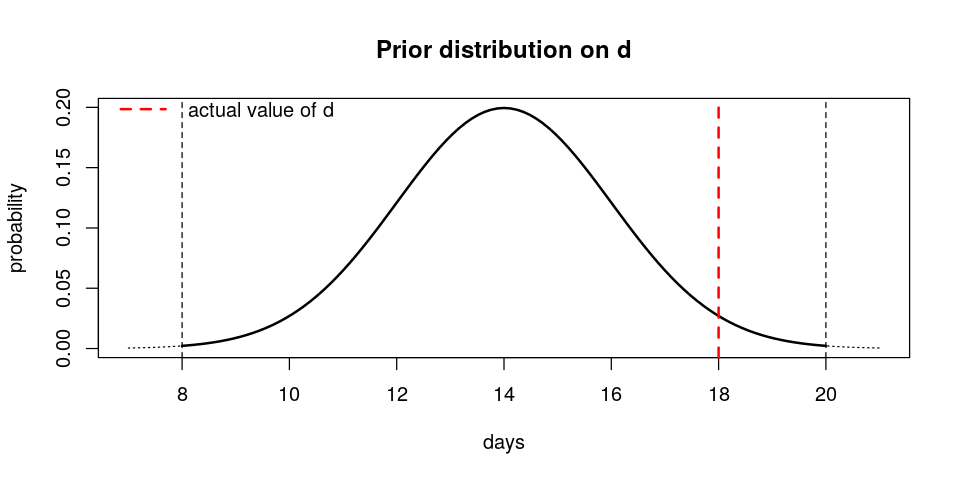In this particular case where we have this additional uncertainty from having $d$ as an unknown, we're going to help the model by setting the lower bound on $\delta_0$ to 0 since it's clear that the intervention had a positive impact.

In :
model_2ec <- stan_model(model_name = "pattern_2ec", model_code = "
data {
int<lower = 1> T; // known time of intervention
int<lower = 1> N; // number observations in series
real y[N];        // observations
}
parameters {
real<lower = -1, upper = 1> phi1;
real<lower = -1, upper = 1> theta1;
real<lower = 0> delta0;
real<lower = 0> sigma;
real<lower = 1> d; // days the change from intervention lasts
}
model {
vector[N] nu;      // prediction at time t
vector[N] z;       // effect of intervention at t
vector[N] epsilon; // diff between pred & obs at t

// priors:
phi1 ~ normal(0, 1);
theta1 ~ normal(0, 1);
delta0 ~ normal(0, 5);
sigma ~ cauchy(0, 5);
d ~ normal(14, 2);

// likelihood:
z = 0;
epsilon = 0;
for (t in 2:N) {
if ((t >= T) && (t < (T + d))) {
z[t] = delta0;
} else {
z[t] = 0;
}
nu[t] = (phi1 * y[t - 1]) + (theta1 * epsilon[t - 1]) + z[t];
epsilon[t] = y[t] - nu[t];
}
epsilon ~ normal(0, sigma);
}")

In :
tic()
fit_2eca <- vb(model_2ec, data = list(T = T, N = N, y = y_2))
toc()
compare(fit_2eca, c(phi1, theta1, delta0, sigma, d), pars = c("phi1", "theta1", "delta0", "sigma", "d"))

Chain 1: ------------------------------------------------------------
Chain 1: EXPERIMENTAL ALGORITHM:
Chain 1:   This procedure has not been thoroughly tested and may be unstable
Chain 1:   or buggy. The interface is subject to change.
Chain 1: ------------------------------------------------------------
Chain 1:
Chain 1:
Chain 1:
Chain 1: Gradient evaluation took 6.6e-05 seconds
Chain 1: 1000 transitions using 10 leapfrog steps per transition would take 0.66 seconds.
Chain 1:
Chain 1:
Chain 1: Iteration:   1 / 250 [  0%]  (Adaptation)
Chain 1: Iteration:  50 / 250 [ 20%]  (Adaptation)
Chain 1: Iteration: 100 / 250 [ 40%]  (Adaptation)
Chain 1: Iteration: 150 / 250 [ 60%]  (Adaptation)
Chain 1: Iteration: 200 / 250 [ 80%]  (Adaptation)
Chain 1: Success! Found best value [eta = 1] earlier than expected.
Chain 1:
Chain 1: Begin stochastic gradient ascent.
Chain 1:   iter             ELBO   delta_ELBO_mean   delta_ELBO_med   notes
Chain 1:    100        -1099.594             1.000            1.000
Chain 1:    200         -966.986             0.569            1.000
Chain 1:    300         -486.536             0.708            0.987
Chain 1:    400         -449.167             0.552            0.987
Chain 1:    500         -447.103             0.442            0.137
Chain 1:    600         -452.099             0.371            0.137
Chain 1:    700         -449.644             0.318            0.083
Chain 1:    800         -446.773             0.279            0.083
Chain 1:    900         -451.716             0.250            0.011
Chain 1:   1000         -447.638             0.226            0.011
Chain 1:   1100         -444.000             0.126            0.011
Chain 1:   1200         -442.544             0.113            0.009   MEDIAN ELBO CONVERGED
Chain 1:
Chain 1: Drawing a sample of size 1000 from the approximate posterior...
Chain 1: COMPLETED.
0.153 sec elapsed

termestimatestd.errorconf.95actual
phi1 -0.690 0.0455 (-0.77, -0.60)-0.7
theta1 0.577 0.0431 (0.49, 0.66) 0.6
delta0 9.060 0.8168 (7.61, 10.69) 10.0
sigma 2.160 0.1297 (1.92, 2.42) 2.0
d 15.661 2.6207 (10.97, 20.90)18.0
In :
tic()
fit_2ecb <- sampling(model_2ec, data = list(T = T, N = N, y = y_2))
toc()
compare(fit_2ecb, c(phi1, theta1, delta0, sigma, d), pars = c("phi1", "theta1", "delta0", "sigma", "d"))

Warning message:
“There were 3019 transitions after warmup that exceeded the maximum treedepth. Increase max_treedepth above 10. See
http://mc-stan.org/misc/warnings.html#maximum-treedepth-exceeded”Warning message:
“Examine the pairs() plot to diagnose sampling problems
”
45.496 sec elapsed

termestimatestd.errorconf.95actual
phi1 -0.753 0.0631 (-0.85, -0.62)-0.7
theta1 0.662 0.0992 (0.45, 0.83) 0.6
delta0 9.754 0.6747 (8.46, 11.10) 10.0
sigma 1.845 0.0884 (1.68, 2.02) 2.0
d 18.359 0.2721 (18.00, 18.89)18.0